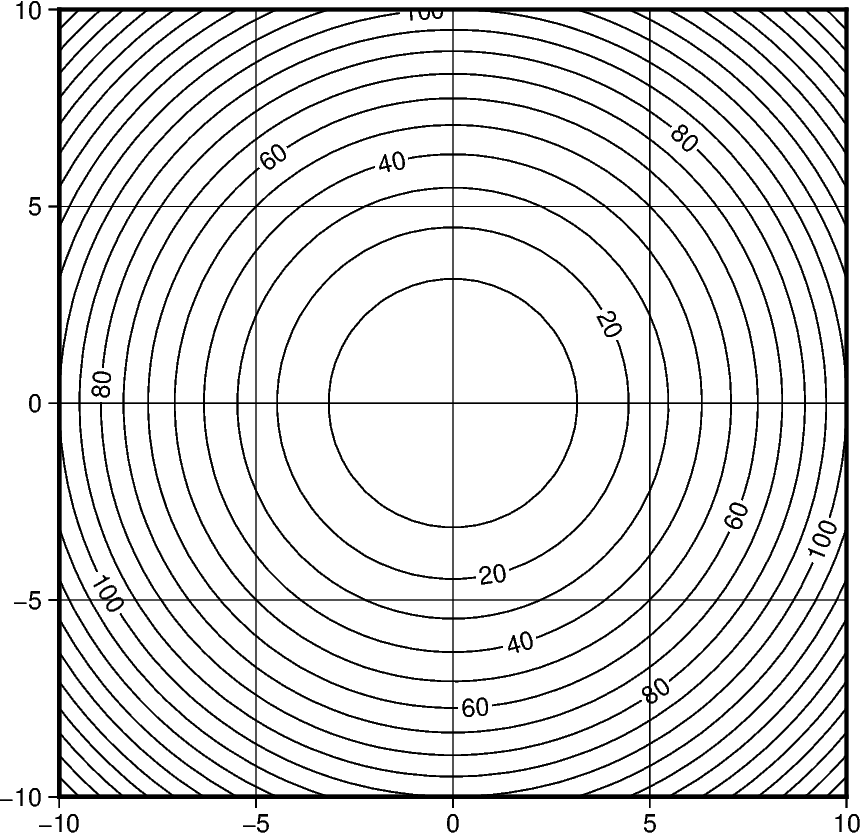Contours

The pygmt.Figure.contour method can plot contour lines from a table of points by direct triangulation. The data for the triangulation can be provided using one of three methods:

1. x, y, z 1d numpy.ndarray data columns.

2. data 2d numpy.ndarray data matrix with 3 columns corresponding to x, y, z.

3. data path string to a file containing the x, y, z in a tabular format.

The parameters levels and annotation set the intervals of the contours and the annotation on the contours respectively.

In this example we supply the data as 1d numpy.ndarray with the x, y, and z parameters and draw the contours using a 0.5p pen with contours every 10 z values and annotations every 20 z values.Out:

<IPython.core.display.Image object>

import numpy as np
import pygmt

# build the contours underlying data with the function z = x^2 + y^2
X, Y = np.meshgrid(np.linspace(-10, 10, 50), np.linspace(-10, 10, 50))
Z = X ** 2 + Y ** 2
x, y, z = X.flatten(), Y.flatten(), Z.flatten()

fig = pygmt.Figure()
fig.contour(
region=[-10, 10, -10, 10],
projection="X10c/10c",
frame="ag",
pen="0.5p",
# pass the data as 3 1d data columns
x=x,
y=y,
z=z,
# set the contours z values intervals to 10
levels=10,
# set the contours annotation intervals to 20
annotation=20,
)
fig.show()

Total running time of the script: ( 0 minutes 1.002 seconds)

Gallery generated by Sphinx-Gallery